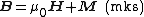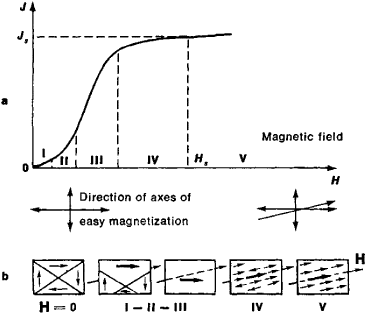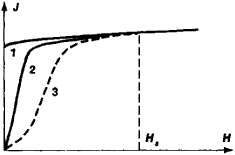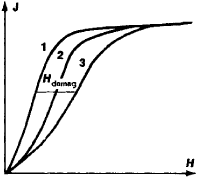# Magnetization

Also found in: Dictionary, Thesaurus, Medical, Wikipedia.

## Magnetization

The process of becoming magnetized; also the property and in particular the extent of being magnetized. Magnetization has an effect on many of the physical properties of a substance. Among these are electrical resistance, specific heat, and elastic strain. See Magnetocaloric effect, Magnetoresistance, Magnetostriction

The magnetization M of a body is caused by circulating electric currents or by elementary atomic magnetic moments, and is defined as the magnetic moment per unit volume of such currents or moments. In the mks (SI) system of units, M is measured in webers per square meter. For M , 1 weber/m2 = 104/4&pgr; gauss.

The magnetic induction or magnetic flux density B is given by the equationbelow, where B and M are in webers/m2, H , the applied magnetic field, is in ampere-turns/m, and μ0, the permeability of free space, is defined as 4&pgr; × 10-7 henry/m, that is, webers/(ampere-turn)(m). See Electrical units and standards

The topic of magnetization is generally restricted to materials exhibiting spontaneous magnetization, that is, magnetization in the absence of H . All such materials will be referred to as ferromagnets, including the special category of ferrimagnets. A ferromagnet is composed of an assemblage of spontaneously magnetized regions called domains. Within each domain, the elementary atomic magnetic moments are essentially aligned, that is, each domain may be envisioned as a small magnet. An unmagnetized ferromagnet is composed of numerous domains, oriented in some fashion.

The process of magnetization in an applied field H consists of growth of those domains oriented most nearly in the direction of H at the expense of others, followed by rotation of the direction of magnetization against anisotropy forces. See Ferromagnetism

On removal of the field H , some magnetization will remain, called the remanence M r.

Curves, sometimes called B-H curves, are used to describe magnetic materials. They are plotted with H as abscissa and with either M or B as ordinate. In the illustration, B r is the remanent induction ( B r = M r); H c is the coercive force, or reverse field required to bring the induction B back to zero; and M s is the saturation magnetization, or magnetization when all domains are aligned. The saturation magnetization is equal to the spontaneous magnetization of a single domain, except that it is possible to increase this magnetization slightly by application of an extremely large field. Saturation magnetization is temperature dependent, and disappears completely above the Curie temperature Tc where a ferromagnet changes into a paramagnet. See Curie temperature

The irreversible nature of magnetization is shown most strikingly by the fact that the path of demagnetization does not retrace the path of magnetization—path 2 of the illustration does not retrace path 1. There is a tendency for the magnetization to show hysteresis, that is, to lag behind the applied field, and the loop of the illustration is called a hysteresis loop.

McGraw-Hill Concise Encyclopedia of Physics. © 2002 by The McGraw-Hill Companies, Inc.
The following article is from The Great Soviet Encyclopedia (1979). It might be outdated or ideologically biased.

## Magnetization

a characteristic of the magnetic state of a macroscopic physical body; in the case of a homogeneously magnetized body, the magnetization is defined as the magnetic moment J per unit volume of the body: J = M/V, where M is the magnetic moment and V is the volume of the body. In the case of an inhomogeneously magnetized body, the magnetization is defined for each point of the body (or, more accurately, for each physically small volume dV): J = dM/dV, where dM is the magnetic moment of the volume dV. In the International System of Units the unit of magnetization is the ampere per meter (1 A/m is the magnetization for which 1 m3 of matter has a magnetic moment of 1 A • mJ), and in the centimeter-gram-second system the unit is the erg/(gauss • cm3); 1 erg/ (gauss • cm3) = 103 A/m.

The magnetization of bodies depends on the external magnetic field and the temperature. In ferromagnets the dependence of J on the intensity H of the external field is expressed by the magnetization curve. In isotropic substances the direction of J coincides with that of H, while in anisotropic substances the directions of J and H generally differ.

### REFERENCES

Vonsovskii, S. V. Magnetizm. Moscow, 1971.
Kirenskii, L. V. Magnetizm, 2nd ed. Moscow, 1967.

## Magnetization

a process that takes place in a ferromagnet that is exposed to an external magnetic field; this process leads to an increase in the intensity of magnetization of the ferromagnet in the direction of the field.

In a state of complete demagnetization, a ferromagnetic specimen consists of small domains having volumes of 10-9-10-6cm3 (occasionally as large as 10 -3 cm3), each of which is magnetized to saturation JS; however, the vectors of spontaneous magnetization of the domains Js are arranged in such a way that the total magnetic moment of the specimen is J = 0.

Magnetization consists in the reorientation of the magnetization vectors of the domains in the direction of an applied field and involves the paraprocess and the processes of displacement and rotation.

The process of displacement in a polydomain ferromagnet consists in the shifting of the boundaries between the domains. The volume of domains whose vectors Js form the smallest angle with the direction of the intensity of magnetic field H increases in the process at the expense of adjacent domains with an energetically less favorable orientation JS with respect to the field. During displacement of the domain boundaries, the domains may change their shape, size, and intrinsic energy. In some cases, these factors promote the displacement process, and in other cases, they impede it. Displacement and magnetization are usually halted when a boundary encounters nonuniformities in the structure of the ferromagnet such as impurity atoms, dislocations, and microcracks. In order for the displacement to resume, the magnitude of H, the temperature, or the pressure must be changed once again.

The process of rotation consists in the turning of the vectors Js in the direction of the field H. The magnetic anisotropy of the ferromagnet is a cause of possible halting or acceleration of the rotation process. (The vectors Js of the domains are initially directed along the axes of easy magnetization, which, in general, are not in the direction of H. When Js lies exactly in the direction of H, technical magnetic saturation, which is equal to the value of Js of the ferromagnet at a given temperature, is achieved.Figure 1. (a) Curve of initial magnetization: (I) region of reversible magnetization, (II) the Rayleigh region, (III) region of maximum permeability, (IV) region near saturation, (V) paraprocess region; (b) schematic diagram of magnetization processes in a polydomain ferromagnet

The paraprocess consists in the ordering, along the field, of the elementary magnetic moments, which may have been deflected from the direction of Js in the domains because of the disorienting effect of the thermal motion. In this case, the value of the magnetization J of the ferromagnet approaches the value of the magnetization at absolute zero. In most cases, the paraprocess produces a very small increase in magnetization; the magnetization of ferromagnets is, therefore, determined primarily by the processes of displacement and rotation.Figure 2. Anhysteretic magnetization curve: theoretical (1) and experimental (2). The curve of initial magnetization (3) is presented for comparison. The slope of the experimental anhysteretic curve is due to nonuniformities of the material, such as voids and cracks, on which internal demagnetizing fields are formed.

If a ferromagnet is magnetized from the state of complete demagnetization (J = H = 0) by a monotonic and slow increase of the field, then the function obtained J(H) is called the initial magnetization curve. This curve is usually divided into five segments (Figure 1). Segment I is the region of initial, or reversible, magnetization, where J = XαH. Elastic displacement of domain boundaries (with a constant initial magnetic susceptibility ξα) is the principal process that takes place in this region. The Rayleigh region (II) is characterized by a quadratic dependence of J on H; in this region, the magnitude of ξ increases linearly with H. In the Rayleigh region, magnetization takes place through displacement processes: a reversible process that is linearly dependent on H and an irreversible process that is quadratically dependent on H. The region of highest permeability (III) is characterized by a rapid increase in J, which is associated with the irreversible displacement of the interdomain boundaries (Bloch walls). Along this segment, magnetization takes place in jumps (the Barkhausen effect). In the region near saturation (IV), rotational processes play the primary role. Segment V is the paraprocess region.Figure 3. Magnetization curves of ferromagnetic specimens of different lengths and shapes: (1) toroid, (2) long, thin specimen, (3) short, thick specimen; (Hdemag,) internal demagnetizing field, which depends on shape of specimen

If H begins to decrease after the state of magnetic saturation Js is reached (in a field Hs), then J will also decrease; the value of J, however, will follow a curve that lies above the initial magnetization curve (the phenomenon of magnetic hysteresis). Hysteresis phenomena also appear during magnetization and hamper the increase in J with increasing field intensity; when they are eliminated, the value of J, even in weak fields, approaches Js, differing from it by an amount that is due to rotational processes (Figure 2). The contributions of displacement and rotational processes to the net magnetization of a ferromagnetic specimen on different segments of the magnetization curves depend on the magnetic texture of the specimen, the presence of defects in the crystal lattice, the shape of the specimen, and other factors. A significant influence of the shape of the specimen on the trend of the magnetization curve (Figure 3) is due to the action of a demagnetizing factor.

### REFERENCES

Vonsovskii, S. V. Magnetizm. Moscow, 1971.
Kirenskii, L. V. Magnetizm, 2nd ed. Moscow, 1967.
Kifer, I. I. Ispytaniia ferromagnitnykh materialov, 3rd ed. Moscow, 1969.
The Great Soviet Encyclopedia, 3rd Edition (1970-1979). © 2010 The Gale Group, Inc. All rights reserved.

## magnetization

[‚mag·nəd·ə′zā·shən]
(electromagnetism)
The property and in particular, the extent of being magnetized; quantitatively, the magnetic moment per unit volume of a substance. Also known as magnetic dipole density; magnetization intensity.
The process of magnetizing a magnetic material.
McGraw-Hill Dictionary of Scientific & Technical Terms, 6E, Copyright © 2003 by The McGraw-Hill Companies, Inc.
References in periodicals archive ?
Magnetization measurements, fluorescence data, FTIR spectra and SEM images reveal the following results; (i) the fluorescence intensity of the POH molecules are quenched considerably in the collapsed state of the gel including especially high Co content (0.3mol/1).
MRAM that uses the "spin-torque-transfer" effect to reverse the direction of magnetization of an element by passing current through it.
1.5T) and recovery of longitudinal magnetization is halted by every refocusing 180 degree RF pulse.
The magnetocrystalline anisotropy energy is minimum for domains located parallel to certain crystallographic directions, which are often called "easy directions of magnetization".
The data gathered from the high and low magnetization signals combine to provide the decoupled signal.
A very useful event for dating Late Paleozoic magnetization is the end of the Kiaman Reversed Epoch at 262 Ma (Opdyke and others 2000).
* Zero-field-cooling magnetization (ZFCM), where the bulk superconductor is firstly cooled to Topwithout any background magnetic field, then such a field is applied and removed; and
The goal of the work is analysis of inhomogeneous magnetization of long cylindrical permalloy 50N cores by a uniform constant magnetic field and the influence of length and field level on their magnetic moment.
In a recently released study, the chemists discovered that light can induce magnetization in certain semiconductors--the standard class of materials at the heart of all computing devices today.
As seen from Figure 2, for the [Fe.sub.65][Al.sub.35] and [Fe.sub.65][Al.sub.30][Ga.sub.5] alloys, the magnetization curves do not reach saturation in external magnetic fields up to [H.sub.ext] = 5.0 T.
Direct visualization of magnetization distribution has provided much information about the basic characteristics of magnetic materials, which has promoted the development and improvement of magnetic devices.

Site: Follow: Share:
Open / Close